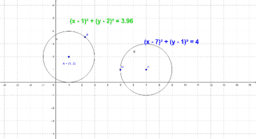•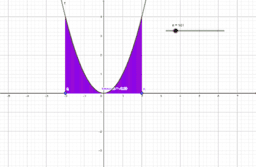### definite integral

Activity

•### definite integral as a sum of areas

Activity

•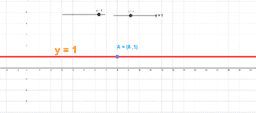### lesson 4 horizontal line

Activity

•### Copy of Lesson No.4 horizontal line

Activity

•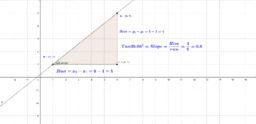### slope of a line

Activity

•### Lsesson 2 distance formula

Activity

•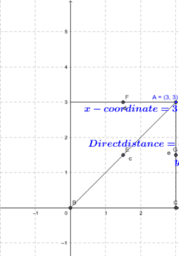### coordinate of a point

Activity

•### coordinate of a point

Activity

•### Lesson No.1 coordinate points dynamically understanding

Activity

•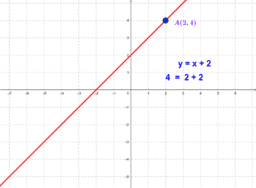### Lesson No.5 verification of a point on a line

Activity

•### Lesson No.7the concept of two intercepts form dynamically

Activity

•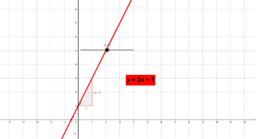### Lesson No.6 the role of b in equation of a line

Activity

•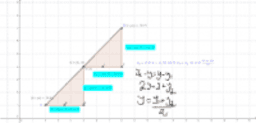### Lesson No14 Mid point formula Process

Activity

•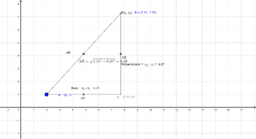### distance formula throughprocess and validation

Activity

•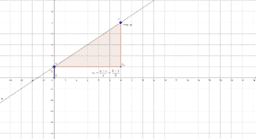### LESSON 5A

Activity

•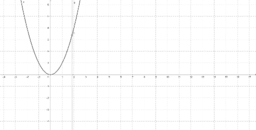### Lesson No19 Function Behavior

Activity

•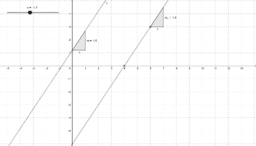### Lesson No18 Line Parralel to a fixed line

Activity

•### Lesson No.18 find perpendicular line to a given line

Activity

•### Lesson No.17point of intersection and concurrency

Activity

•### Lesson No15 Ratio formulaArithmetization

Activity

•### Lesson No.16 Ratio formula Process arithmetization

Activity

•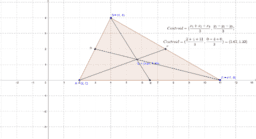### Lesson No13 Mid Point and Centroid

Activity

•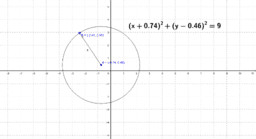### LessonNo12 analysis of circle

Activity

•